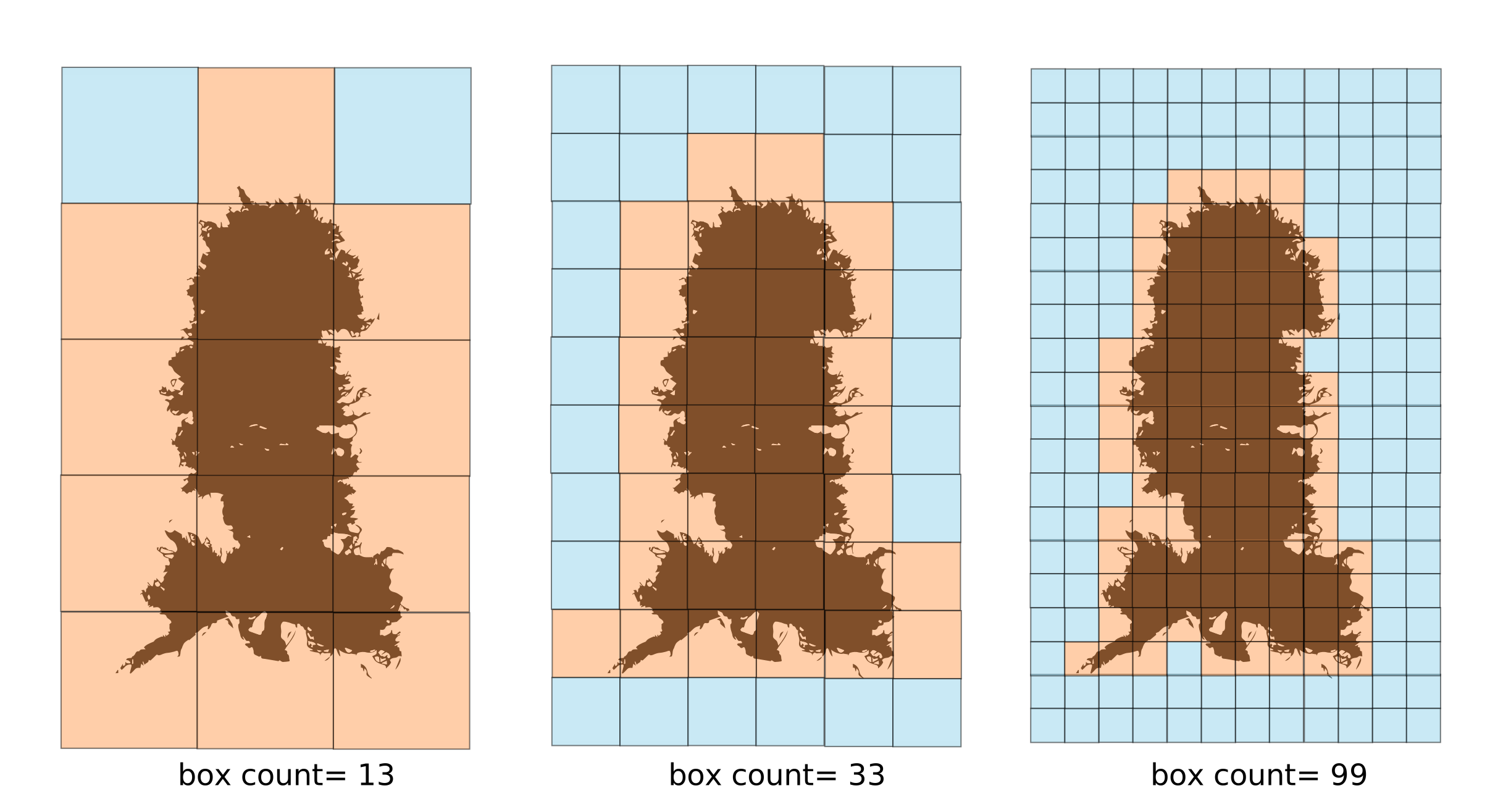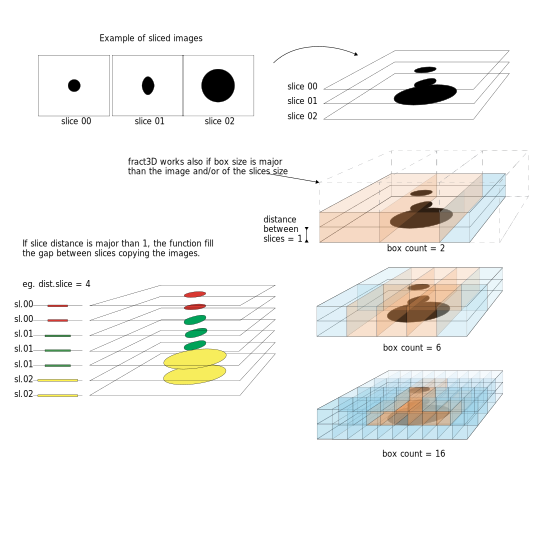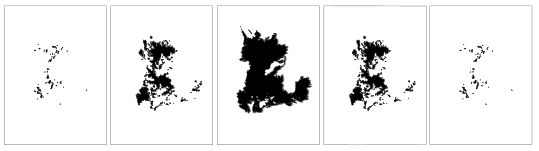Calculates the fractal dimension of 2D and 3D (sliced) images

2021-01-29

The package fractD contains two fuctions fract2D and fract3D that allow to estimate the fractal dimension (D) of 2D and 3D images. Fractal dimension is estimated by the method of box-counting. Box-counting method is useful to calculate the fractal dimension of various sets of any dimension and patterns with or without self-similarity (Klinkenberg, 1994). The method overlay a series of squares (for fract2D) or cubes (for fract3D) of different sizes. Then, for each size step the functions keep track of the number of squares or cubes occupied by the black area into the image. Finally, the fractal dimension (D) is estimated by linear regression of log(n°boxes) on log(box.size).

The following illustration show the rational behind the function fract2D:Here an example of applying fract2D to the following image:library(fractD)
## basic example code for fract2D
fct2D <- fract2D(dir = "examples/source.dir", box.size = c(1,2,4,8,16,32,64,128,256,512))

## the following code saves the data in a file named "es3D.rds" into the "output"" folder
## not run ##
##fct2D <- fract2D(dir = "examples/source.dir", box.size = c(1,2,4,8,16,32,64,128,256,512), save.dir = "examples/output/", save.name = "es2D")
# the function create a list with two objects:
fct2D$D # Estimated fractal dimension #> id D #> 1 fig_1 1.7669 fct2D$raw.dat # Raw data from which fractal dimension was calculated
#>       id box.size    box
#> 1  fig_1        1 328905
#> 2  fig_1        2  86845
#> 3  fig_1        4  23155
#> 4  fig_1        8   6207
#> 5  fig_1       16   1681
#> 6  fig_1       32    462
#> 7  fig_1       64    135
#> 8  fig_1      128     44
#> 9  fig_1      256     17
#> 10 fig_1      512      6

The following illustration show the rational behind the function fract3D:Here an example of applying fract3D to the following image:#library(fractD)
## basic example code for fract3D
# save.dir and save.name provide optional
fct3D <- fract3D(dir = "examples/source.dir", dist.slice = 10, box.size = c(4,8,16,32,128,256,512))

# the following code saves the data in a file named "es3D.rds" into the "output"" folder
## not run ##
## fct3D <- fract3D(dir = "examples/source.dir", dist.slice = 1, box.size = c(1,2,4,8,16,32,64,128,256,512), save.dir = "examples/output/", save.name = "es3D")
# the function create a list with two objects:
fct3D$D # Estimated fractal dimension #> id D #> 1 es_3D_img 2.0599 fct3D$raw.dat # Raw data from which fractal dimension was calculated
#>          id box.size    box
#> 1 es_3D_img        4 120164
#> 2 es_3D_img        8  22632
#> 3 es_3D_img       16   4066
#> 4 es_3D_img       32    736
#> 5 es_3D_img      128     43
#> 6 es_3D_img      256     17
#> 7 es_3D_img      512      6